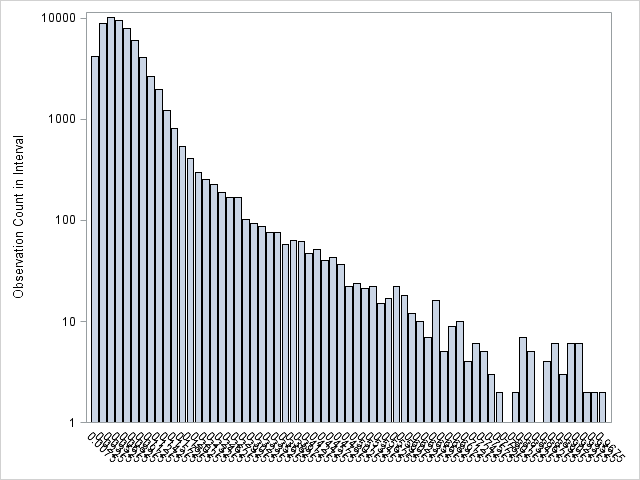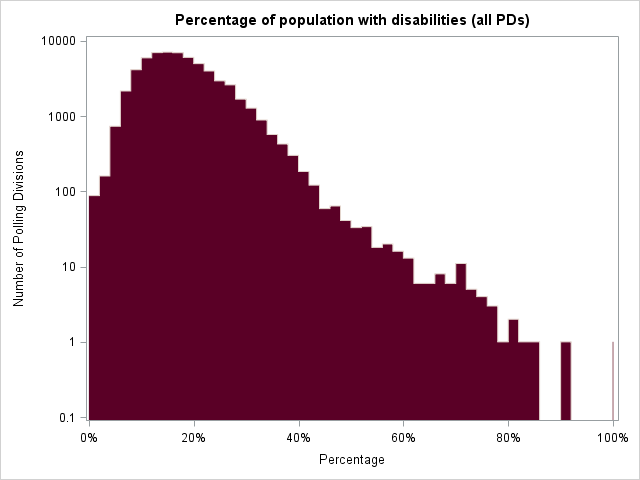## How to plot a histogram with a log scale y axis when baseline = doesn't work

Hello,

I'm trying to create a histogram where both the x and y axes are on the log scale so that I can determine whether my variable is power-law distributed. Essentially, I only have one variable which is a count variable of the number of "likes" for each comment on a Nike YouTube video. Here is my code:

title 'Histogram of Logged "Likes" for the Nike YouTube Video';
proc sgplot data = twopart;
where Video = "Nike";
vbar loglikes / groupdisplay = cluster baseline=.0000001;
yaxis type = log logbase = 10;
run;

I simply log transformed my variable to get the x axis on the log scale, but I'm having trouble transforming the y axis. I've read a few other posts that say the best thing to do when your axis contains zeros is to use the baseline = option, but I keep getting an error message that says:

2664 vbar loglikes / groupdisplay = cluster baseline=.0000001;
--------
22
76
ERROR 22-322: Syntax error, expecting one of the following: ;, ALPHA, ATTRID, BARWIDTH,
CATEGORYORDER, CLUSTERWIDTH, DATALABEL, DATALABELATTRS, DATALABELFITPOLICY,
DATALABELPOS, DATASKIN, DISCRETEOFFSET, FILL, FILLATTRS, FREQ, GROUP,
GROUPDISPLAY, GROUPORDER, LEGENDLABEL, LIMITATTRS, LIMITS, LIMITSTAT,
MISSING, NAME, NOFILL, NOOUTLINE, NOSTATLABEL, NUMSTD, OUTLINE,
OUTLINEATTRS, RESPONSE, SPLITCHAR, SPLITCHARNODROP, STAT, TIP, TIPFORMAT,
TIPLABEL, TRANSPARENCY, URL, WEIGHT, X2AXIS, Y2AXIS.
ERROR 76-322: Syntax error, statement will be ignored.

I'm using SAS version 9.4.

I would greatly appreciate any help. Thank you!

13 REPLIES 13DanH_sas
SAS Super FREQ

## Re: How to plot a histogram with a log scale y axis when baseline = doesn't work

The BASELINE option was introduced for bar charts in SAS 9.4m1. Do you have SAS 9.4 without any maintenance updates? What does the following give you?

%put &sysvlong;

## Re: How to plot a histogram with a log scale y axis when baseline = doesn't work

Thank you for your quick response.

I get the following:

9.04.01M0P061913DanH_sas
SAS Super FREQ

## Re: How to plot a histogram with a log scale y axis when baseline = doesn't work

Yes, you have the first release of SAS 9.4, without any maintenance updates.

## Re: How to plot a histogram with a log scale y axis when baseline = doesn't work

From the variable name (loglikes), it sounds like you have already transformed the response variable. If so, you can use a regular linear Y axis and simply use LABEL='log likes' to indicate that the axis is on a log scale.

If you use a log axis on data that has already been log-transformed, you will be viewing doubly-logged data.

## Re: How to plot a histogram with a log scale y axis when baseline = doesn't work

Yes, I would like to create a doubly-logged plot. I'm trying to determine whether my variable "likes" follows a power-law distribution, so I've taken the log of "likes" and now I need to view it on a logged y axis.

My understanding is that I'd be able to create a log-log plot if I could use the baseline = log option, but my version of SAS isn't a candidate for maintenance updates.

## Re: How to plot a histogram with a log scale y axis when baseline = doesn't work

I don't advocate using a doubly-logged Y variable, but if you choose to do so, you can use the data step to compute

LogLogLikes = log10(log10(likes));

and then use a linear scale on the vertical bar height.

Basically, you can transform the Y any way you want. You also need to bin the data yourself (which I assume you've already don) and then run

VBAR xBin / response=LogLogLikes;

This will emulate the histogram with a transformed Y axis.

## Re: How to plot a histogram with a log scale y axis when baseline = doesn't work

Hi,

I have a similar problem.

I have obtained the histogram bins from PROC UNIVARIATE, and my goal is to plot the output dataset as an histogram with a Log Y axis using PROC SGPLOT with VBAR and BASELINE=1

The issue is that VBAR adds its own ticks and labels, and the resulting plot is illegible:So my question is the following: Is there a way to prevent VBAR to show the bar labels?DanH_sas
SAS Super FREQ

## Re: How to plot a histogram with a log scale y axis when baseline = doesn't work

To get rid of the axis labels, just add the following statement to SGPLOT:

xaxis display=none;

## Re: How to plot a histogram with a log scale y axis when baseline = doesn't work

Oh weird I thought I had tried it, thanks!

## Re: How to plot a histogram with a log scale y axis when baseline = doesn't work

I have implemented a workaround that generates a histogram-like plot.

It works like this:

• You first obtain a dataset with bin data from PROC UNIVARIATE
• If any bin is equal to zero, you add a small nonzero value (e.g. 1e-9)
• You use PROC SGPLOT to plot a STEP line, which will be the outline of your "histogram"
• In the same PROC SGPLOT block, you use a BAND statement to fill the area below the outline
• You give the y axis a lower limit of 1e-1

The result looks like this, with some minor tweaks it can pass as a histogram:And here's the macro that does it:

``````%macro plot_logy_histo(inData=, var=,  title=, debug=0);

%local bindat;

/* create dataset with bin contents */
proc univariate data=&inData. noprint;
histogram &var. / vscale=count outhistogram=_DATA_(label=plot_logy_histo bindat) noplot;
run;
%let bindat=&SYSLAST.;

/* add dummy data to zero bins */
data &bindat.;
set &bindat.;
if _count_ eq 0 then _count_=_count_+1e-9;
run;

proc sgplot data=&bindat. noautolegend;
title "&title.";
yaxis logbase=10 type=log min=1e-1 label="Number of Polling Divisions";
xaxis valuesformat=percentn7. label="Percentage";
step x=_midpt_ y=_count_ / lineattrs=(thickness=0) name = "outline";
band x=_midpt_ upper=_count_ lower=1e-9 / outline fill modelname="outline" fillattrs=(color=&ec_burgundy.);
run;
title;

%if &debug. eq 0 %then %do;
proc delete data=&bindat.;
run;
%end;

%mend;``````

## Re: How to plot a histogram with a log scale y axis when baseline = doesn't work

Why not just replace zero counts with a missing value?

``if _count_ eq 0 then _count_=.;``

## Re: How to plot a histogram with a log scale y axis when baseline = doesn't work

I haven't tried that, it should work too. Thanks!

## Re: How to plot a histogram with a log scale y axis when baseline = doesn't work

Actually, setting _count_=. has an unpleasant side effect: if the data for a bin is missing, STEP will interpolate the missing data from the neighbouring bins.

So, I think it's better to add a small nonzero value: this forces STEP to go below the visible y axis range.

Discussion stats
• 13 replies
• 1954 views
• 4 likes
• 4 in conversation